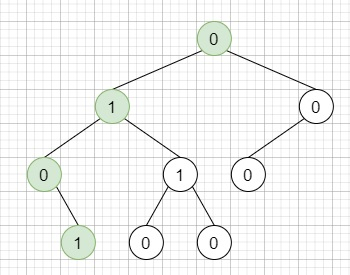# Check If a String Is a Valid Sequence from Root to Leaves Path in a Binary Tree in C++

Suppose we have a binary tree where each path going from the root to any leaf form a valid sequence, we have to check whether a given string is a valid sequence in such binary tree or not.

We will get the given string from the concatenation of an array of integers arr and the concatenation of all values of the nodes along a path results in a sequence

Suppose we have a binary tree like.So, if arr = [0,1,0,1], then the output will be True, as the path 0 -> 1 -> 0 -> 1 is a valid sequence (green color). Other valid sequences will be : 0 -> 1 -> 1 -> 0 , 0 -> 0 -> 0

To solve this, we will follow these steps −

• Define a function solve(), this will take node, an array v, idx initialize it with 0,

• if node is null, then −

• return false

• if idx >= size of v, then −

• return false

• if val of node is not equal to v[idx], then −

• return false

• if node has no children, then −

• return true when idx == size of v

• return solve(left of node, v, idx + 1)

• from the main method do the following −

• return solve(root, arr)

## Example

Let us see the following implementation to get better understanding −

Live Demo

#include <bits/stdc++.h>
using namespace std;
class TreeNode{
public:
int val;
TreeNode *left, *right;
TreeNode(int data){
val = data;
left = NULL;
right = NULL;
}
};
class Solution {
public:
bool solve(TreeNode* node, vector <int>& v, int idx = 0){
if(!node) return false;
if(idx >= v.size()) return false;
if(node->val != v[idx]) return false;
if(!node->left && !node->right){
return idx == v.size() - 1;
}
return solve(node->left, v, idx + 1) || solve(node->right, v, idx + 1);
}
bool isValidSequence(TreeNode* root, vector<int>& arr) {
return solve(root, arr);
}
};
main(){
TreeNode *root = new TreeNode(0);
root->left = new TreeNode(1); root->right = new TreeNode(0);
root->left->left = new TreeNode(0); root->left->right = new
TreeNode(1);
root->right->left = new TreeNode(0);
root->left->left->right = new TreeNode(1);
root->left->right->left = new TreeNode(0); root->left->right->right = new TreeNode(0);
Solution ob;
vector<int> v = {0,1,0,1};
cout << (ob.isValidSequence(root, v));
}

## Input

TreeNode *root = new TreeNode(0);
root->left = new TreeNode(1); root->right = new TreeNode(0);
root->left->left = new TreeNode(0); root->left->right = new
TreeNode(1);
root->right->left = new TreeNode(0);
root->left->left->right = new TreeNode(1);
root->left->right->left = new TreeNode(0); root->left->right->right = new TreeNode(0);

## Output

1